# 零基础入门深度学习(4) - 卷积神经网络

机器学习 深度学习入门## 一个新的激活函数——Relu

Relu函数图像如下图所示：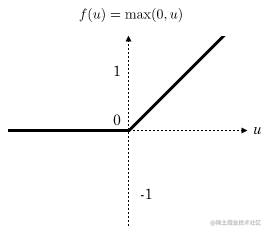Relu函数作为激活函数，有下面几大优势：

• 速度快 和sigmoid函数需要计算指数和倒数相比，relu函数其实就是一个max(0,x)，计算代价小很多。
• 减轻梯度消失问题 回忆一下计算梯度的公式。其中，是sigmoid函数的导数。在使用反向传播算法进行梯度计算时，每经过一层sigmoid神经元，梯度就要乘上一个。从下图可以看出，函数最大值是1/4。因此，乘一个会导致梯度越来越小，这对于深层网络的训练是个很大的问题。而relu函数的导数是1，不会导致梯度变小。当然，激活函数仅仅是导致梯度减小的一个因素，但无论如何在这方面relu的表现强于sigmoid。使用relu激活函数可以让你训练更深的网络。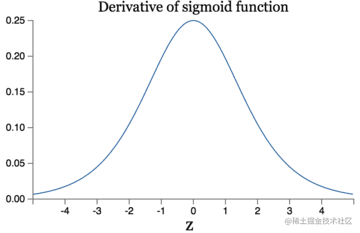• 稀疏性 通过对大脑的研究发现，大脑在工作的时候只有大约5%的神经元是激活的，而采用sigmoid激活函数的人工神经网络，其激活率大约是50%。有论文声称人工神经网络在15%-30%的激活率时是比较理想的。因为relu函数在输入小于0时是完全不激活的，因此可以获得一个更低的激活率。

## 全连接网络 VS 卷积网络

• 参数数量太多 考虑一个输入1000*1000像素的图片(一百万像素，现在已经不能算大图了)，输入层有1000*1000=100万节点。假设第一个隐藏层有100个节点(这个数量并不多)，那么仅这一层就有(1000*1000+1)*100=1亿参数，这实在是太多了！我们看到图像只扩大一点，参数数量就会多很多，因此它的扩展性很差。
• 没有利用像素之间的位置信息 对于图像识别任务来说，每个像素和其周围像素的联系是比较紧密的，和离得很远的像素的联系可能就很小了。如果一个神经元和上一层所有神经元相连，那么就相当于对于一个像素来说，把图像的所有像素都等同看待，这不符合前面的假设。当我们完成每个连接权重的学习之后，最终可能会发现，有大量的权重，它们的值都是很小的(也就是这些连接其实无关紧要)。努力学习大量并不重要的权重，这样的学习必将是非常低效的。
• 网络层数限制 我们知道网络层数越多其表达能力越强，但是通过梯度下降方法训练深度全连接神经网络很困难，因为全连接神经网络的梯度很难传递超过3层。因此，我们不可能得到一个很深的全连接神经网络，也就限制了它的能力。

• 局部连接 这个是最容易想到的，每个神经元不再和上一层的所有神经元相连，而只和一小部分神经元相连。这样就减少了很多参数。
• 权值共享 一组连接可以共享同一个权重，而不是每个连接有一个不同的权重，这样又减少了很多参数。
• 下采样 可以使用Pooling来减少每层的样本数，进一步减少参数数量，同时还可以提升模型的鲁棒性。

## 卷积神经网络是啥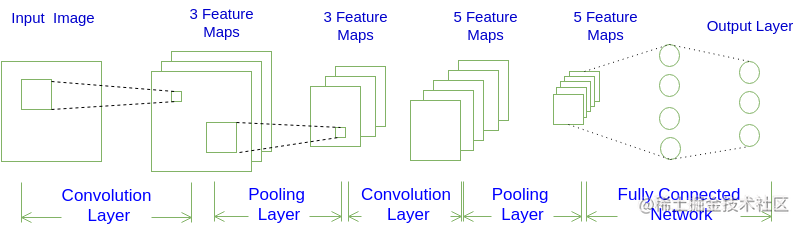### 网络架构

INPUT -> [[CONV]*N -> POOL?]*M -> [FC]*K

INPUT -> CONV -> POOL -> CONV -> POOL -> FC -> FC

INPUT -> [[CONV]*1 -> POOL]*2 -> [FC]*2

## 卷积神经网络输出值的计算

### 卷积层输出值的计算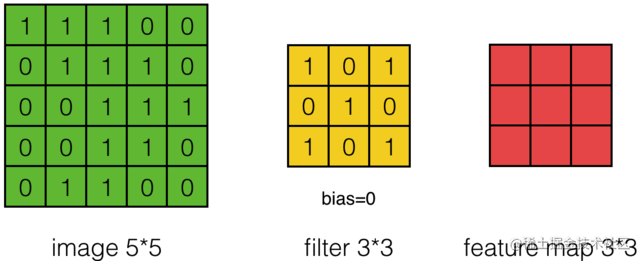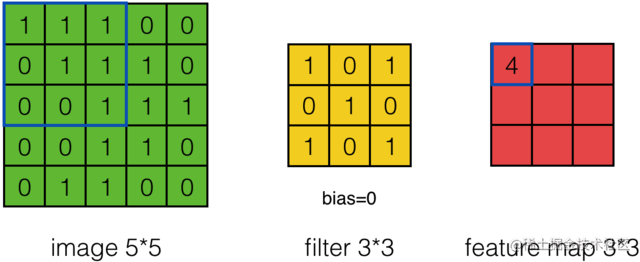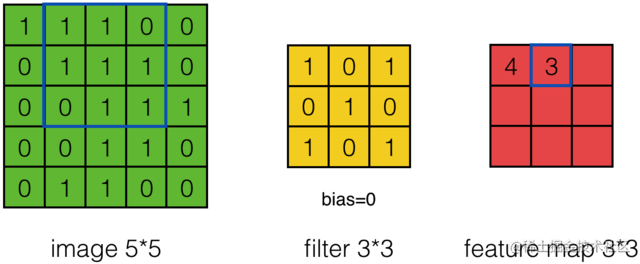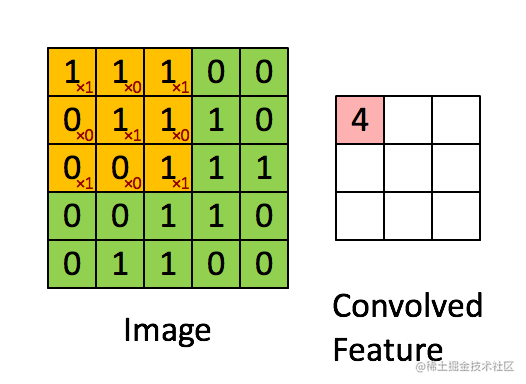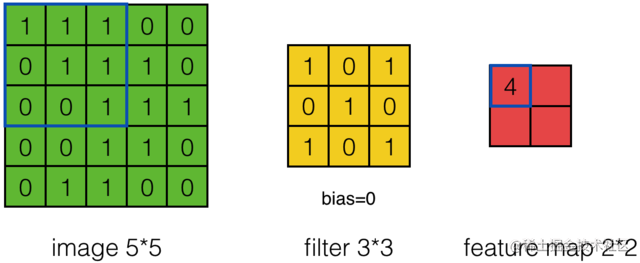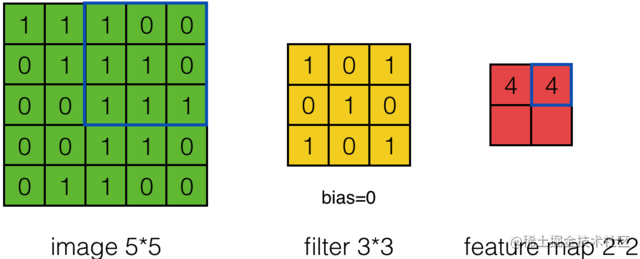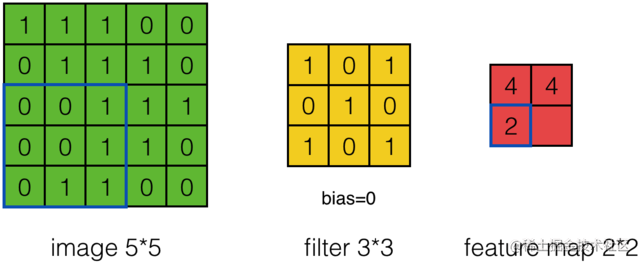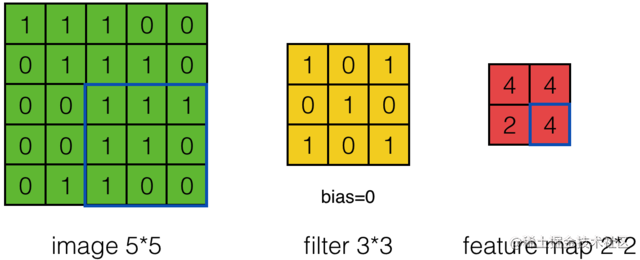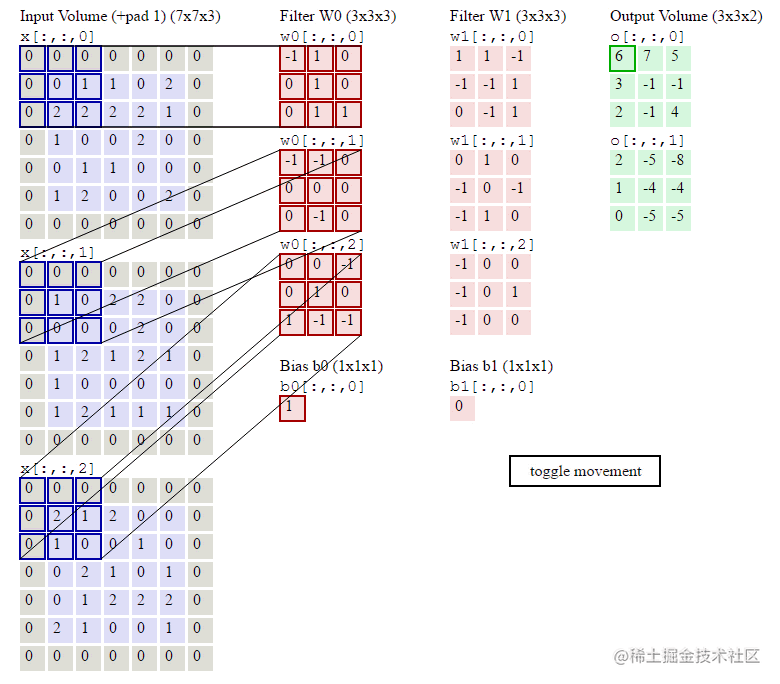#### 用卷积公式来表达卷积层计算,满足条件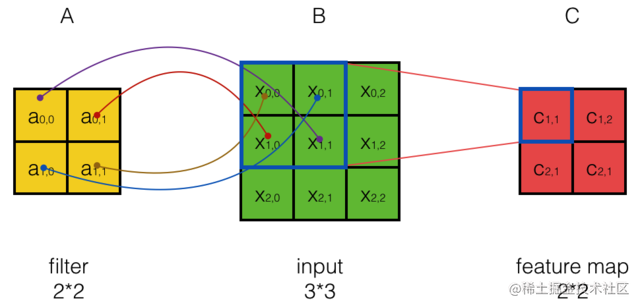### Pooling层输出值的计算

Pooling层主要的作用是下采样，通过去掉Feature Map中不重要的样本，进一步减少参数数量。Pooling的方法很多，最常用的是Max PoolingMax Pooling实际上就是在n*n的样本中取最大值，作为采样后的样本值。下图是2*2 max pooling：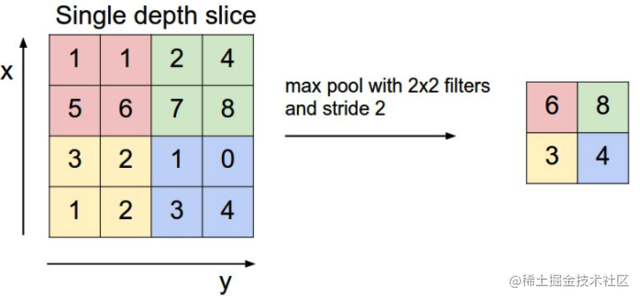## 卷积神经网络的训练

1. 前向计算每个神经元的输出值表示网络的第个神经元，以下同）；
2. 反向计算每个神经元的误差项在有的文献中也叫做敏感度(sensitivity)。它实际上是网络的损失函数对神经元加权输入的偏导数，即3. 计算每个神经元连接权重梯度表示从神经元连接到神经元的权重），公式为，其中，表示神经元的输出。

### 卷积层的训练

#### 卷积层误差项的传递

##### 最简单情况下误差项的传递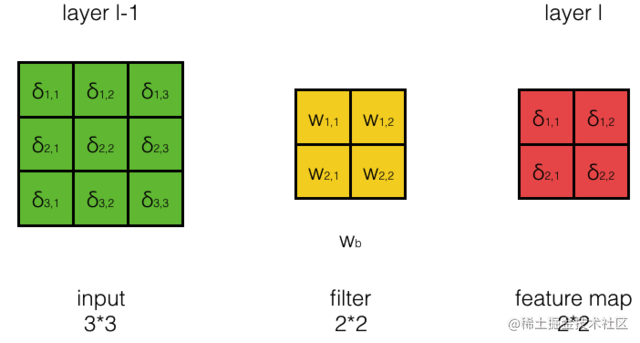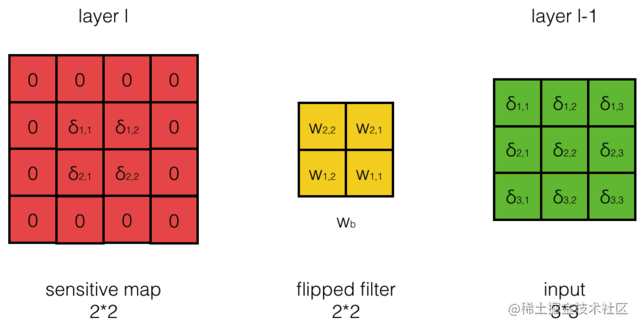##### 卷积步长为S时的误差传递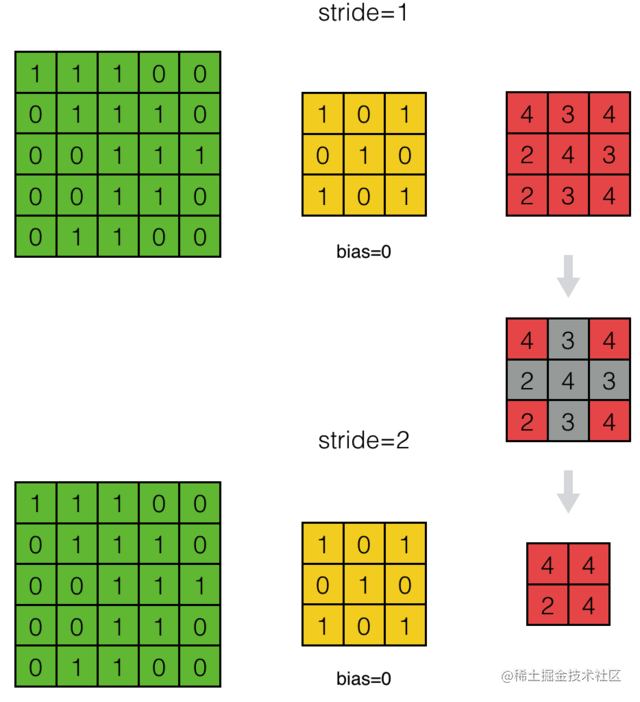##### 输入层深度为D时的误差传递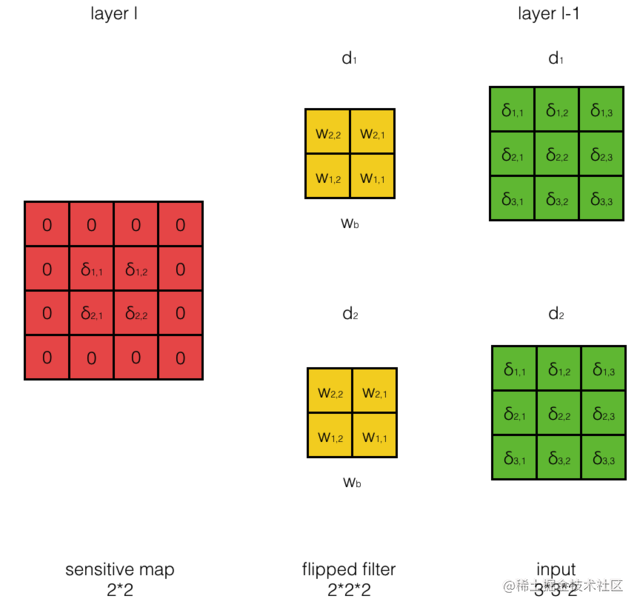##### filter数量为N时的误差传递

filter数量为N时，输出层的深度也为N，第个filter卷积产生输出层的第个feature map。由于第每个加权输入都同时影响了第层所有feature map的输出值，因此，反向计算误差项时，需要使用全导数公式。也就是，我们先使用第个filter对第层相应的第个sensitivity map进行卷积，得到一组N个层的偏sensitivity map。依次用每个filter做这种卷积，就得到D组偏sensitivity map。最后在各组之间将N个偏sensitivity map 按元素相加，得到最终的N个层的sensitivity map：

#### 卷积层filter权重梯度的计算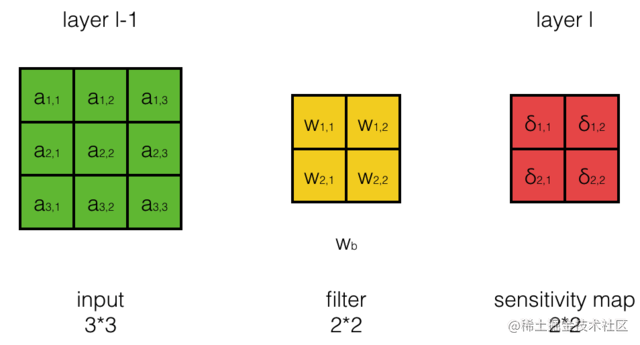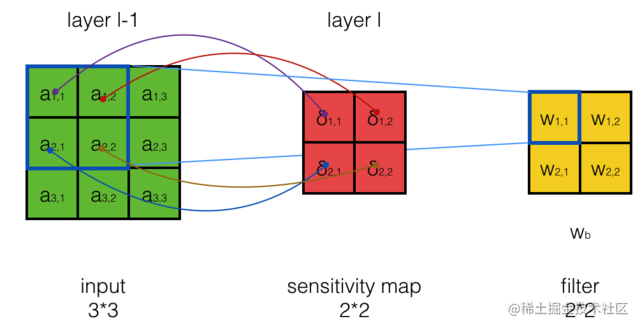### Pooling层的训练

#### Max Pooling误差项的传递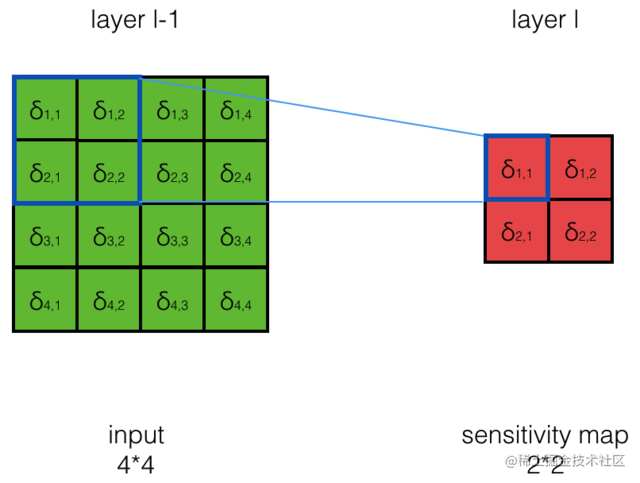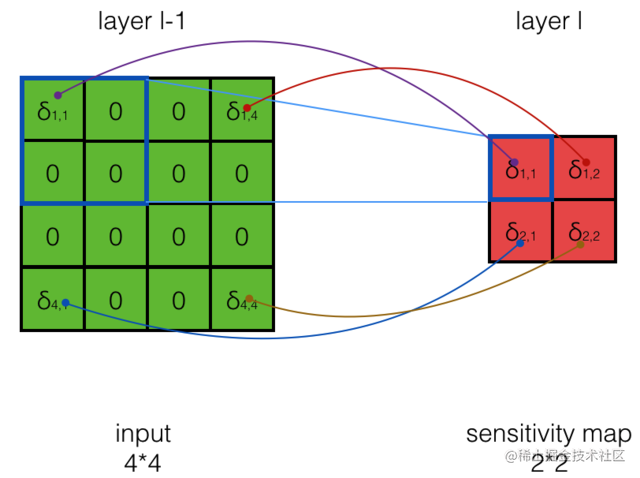#### Mean Pooling误差项的传递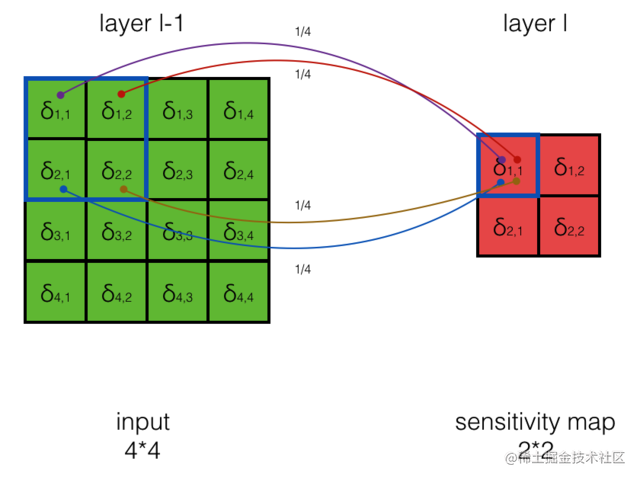## 卷积神经网络的实现

import numpy as np复制代码

### 卷积层的实现

#### 卷积层初始化

class ConvLayer(object):
def __init__(self, input_width, input_height,
channel_number, filter_width,
filter_height, filter_number,
learning_rate):
self.input_width = input_width
self.input_height = input_height
self.channel_number = channel_number
self.filter_width = filter_width
self.filter_height = filter_height
self.filter_number = filter_number
self.stride = stride
self.output_width = \
ConvLayer.calculate_output_size(
stride)
self.output_height = \
ConvLayer.calculate_output_size(
stride)
self.output_array = np.zeros((self.filter_number,
self.output_height, self.output_width))
self.filters = []
for i in range(filter_number):
self.filters.append(Filter(filter_width,
filter_height, self.channel_number))
self.activator = activator
self.learning_rate = learning_rate复制代码

calculate_output_size函数用来确定卷积层输出的大小，其实现如下：

    @staticmethod
def calculate_output_size(input_size,
return (input_size - filter_size +
2 * zero_padding) / stride + 1复制代码

Filter类保存了卷积层的参数以及梯度，并且实现了用梯度下降算法来更新参数。

class Filter(object):
def __init__(self, width, height, depth):
self.weights = np.random.uniform(-1e-4, 1e-4,
(depth, height, width))
self.bias = 0
self.weights.shape)

def __repr__(self):
return 'filter weights:\n%s\nbias:\n%s' % (
repr(self.weights), repr(self.bias))

def get_weights(self):
return self.weights

def get_bias(self):
return self.bias

def update(self, learning_rate):
self.bias -= learning_rate * self.bias_grad复制代码

Activator类实现了激活函数，其中，forward方法实现了前向计算，而backward方法则是计算导数。比如，relu函数的实现如下：

class ReluActivator(object):
def forward(self, weighted_input):
#return weighted_input
return max(0, weighted_input)

def backward(self, output):
return 1 if output > 0 else 0复制代码

#### 卷积层前向计算的实现

ConvLayer类的forward方法实现了卷积层的前向计算（即计算根据输入来计算卷积层的输出），下面是代码实现：

    def forward(self, input_array):
'''
计算卷积层的输出
输出结果保存在self.output_array
'''
self.input_array = input_array
for f in range(self.filter_number):
filter = self.filters[f]
filter.get_weights(), self.output_array[f],
self.stride, filter.get_bias())
element_wise_op(self.output_array,
self.activator.forward)复制代码

# 对numpy数组进行element wise操作
def element_wise_op(array, op):
for i in np.nditer(array,
i[...] = op(i)复制代码

conv函数实现了2维和3维数组的卷积，代码如下：

def conv(input_array,
kernel_array,
output_array,
stride, bias):
'''
计算卷积，自动适配输入为2D和3D的情况
'''
channel_number = input_array.ndim
output_width = output_array.shape
output_height = output_array.shape
kernel_width = kernel_array.shape[-1]
kernel_height = kernel_array.shape[-2]
for i in range(output_height):
for j in range(output_width):
output_array[i][j] = (
get_patch(input_array, i, j, kernel_width,
kernel_height, stride) * kernel_array
).sum() + bias复制代码

# 为数组增加Zero padding
'''
'''
if zp == 0:
return input_array
else:
if input_array.ndim == 3:
input_width = input_array.shape
input_height = input_array.shape
input_depth = input_array.shape
input_depth,
input_height + 2 * zp,
input_width + 2 * zp))
zp : zp + input_height,
zp : zp + input_width] = input_array
elif input_array.ndim == 2:
input_width = input_array.shape
input_height = input_array.shape
input_height + 2 * zp,
input_width + 2 * zp))
zp : zp + input_width] = input_array
return padded_array复制代码

#### 卷积层反向传播算法的实现

1. 误差项传递到上一层。
2. 计算每个参数梯度
3. 更新参数

    def bp_sensitivity_map(self, sensitivity_array,
activator):
'''
计算传递到上一层的sensitivity map
sensitivity_array: 本层的sensitivity map
activator: 上一层的激活函数
'''
# 处理卷积步长，对原始sensitivity map进行扩展
expanded_array = self.expand_sensitivity_map(
sensitivity_array)
# 但这个残差不需要继续向上传递，因此就不计算了
expanded_width = expanded_array.shape
zp = (self.input_width +
self.filter_width - 1 - expanded_width) / 2
# 初始化delta_array，用于保存传递到上一层的
# sensitivity map
self.delta_array = self.create_delta_array()
# 对于具有多个filter的卷积层来说，最终传递到上一层的
# sensitivity map相当于所有的filter的
# sensitivity map之和
for f in range(self.filter_number):
filter = self.filters[f]
# 将filter权重翻转180度
flipped_weights = np.array(map(
lambda i: np.rot90(i, 2),
filter.get_weights()))
# 计算与一个filter对应的delta_array
delta_array = self.create_delta_array()
for d in range(delta_array.shape):
delta_array[d], 1, 0)
self.delta_array += delta_array
# 将计算结果与激活函数的偏导数做element-wise乘法操作
derivative_array = np.array(self.input_array)
element_wise_op(derivative_array,
activator.backward)
self.delta_array *= derivative_array复制代码

expand_sensitivity_map方法就是将步长为S的sensitivity map『还原』为步长为1的sensitivity map，代码如下：

    def expand_sensitivity_map(self, sensitivity_array):
depth = sensitivity_array.shape
# 确定扩展后sensitivity map的大小
# 计算stride为1时sensitivity map的大小
expanded_width = (self.input_width -
self.filter_width + 2 * self.zero_padding + 1)
expanded_height = (self.input_height -
self.filter_height + 2 * self.zero_padding + 1)
# 构建新的sensitivity_map
expand_array = np.zeros((depth, expanded_height,
expanded_width))
# 从原始sensitivity map拷贝误差值
for i in range(self.output_height):
for j in range(self.output_width):
i_pos = i * self.stride
j_pos = j * self.stride
expand_array[:,i_pos,j_pos] = \
sensitivity_array[:,i,j]
return expand_array复制代码

create_delta_array是创建用来保存传递到上一层的sensitivity map的数组。

    def create_delta_array(self):
return np.zeros((self.channel_number,
self.input_height, self.input_width))复制代码

    def bp_gradient(self, sensitivity_array):
# 处理卷积步长，对原始sensitivity map进行扩展
expanded_array = self.expand_sensitivity_map(
sensitivity_array)
for f in range(self.filter_number):
# 计算每个权重的梯度
filter = self.filters[f]
for d in range(filter.weights.shape):
expanded_array[f],
# 计算偏置项的梯度
filter.bias_grad = expanded_array[f].sum()复制代码

    def update(self):
'''
按照梯度下降，更新权重
'''
for filter in self.filters:
filter.update(self.learning_rate)复制代码

#### 卷积层的梯度检查

def init_test():
a = np.array(
[[[0,1,1,0,2],
[2,2,2,2,1],
[1,0,0,2,0],
[0,1,1,0,0],
[1,2,0,0,2]],
[[1,0,2,2,0],
[0,0,0,2,0],
[1,2,1,2,1],
[1,0,0,0,0],
[1,2,1,1,1]],
[[2,1,2,0,0],
[1,0,0,1,0],
[0,2,1,0,1],
[0,1,2,2,2],
[2,1,0,0,1]]])
b = np.array(
[[[0,1,1],
[2,2,2],
[1,0,0]],
[[1,0,2],
[0,0,0],
[1,2,1]]])
cl = ConvLayer(5,5,3,3,3,2,1,2,IdentityActivator(),0.001)
cl.filters.weights = np.array(
[[[-1,1,0],
[0,1,0],
[0,1,1]],
[[-1,-1,0],
[0,0,0],
[0,-1,0]],
[[0,0,-1],
[0,1,0],
[1,-1,-1]]], dtype=np.float64)
cl.filters.bias=1
cl.filters.weights = np.array(
[[[1,1,-1],
[-1,-1,1],
[0,-1,1]],
[[0,1,0],
[-1,0,-1],
[-1,1,0]],
[[-1,0,0],
[-1,0,1],
[-1,0,0]]], dtype=np.float64)
return a, b, cl

'''
梯度检查
'''
# 设计一个误差函数，取所有节点输出项之和
error_function = lambda o: o.sum()

# 计算forward值
a, b, cl = init_test()
cl.forward(a)

# 求取sensitivity map，是一个全1数组
sensitivity_array = np.ones(cl.output_array.shape,
dtype=np.float64)
# 计算梯度
cl.backward(a, sensitivity_array,
IdentityActivator())
# 检查梯度
epsilon = 10e-4
cl.filters.weights[d,i,j] += epsilon
cl.forward(a)
err1 = error_function(cl.output_array)
cl.filters.weights[d,i,j] -= 2*epsilon
cl.forward(a)
err2 = error_function(cl.output_array)
expect_grad = (err1 - err2) / (2 * epsilon)
cl.filters.weights[d,i,j] += epsilon
print 'weights(%d,%d,%d): expected - actural %f - %f' % (
d, i, j, expect_grad, cl.filters.weights_grad[d,i,j])   复制代码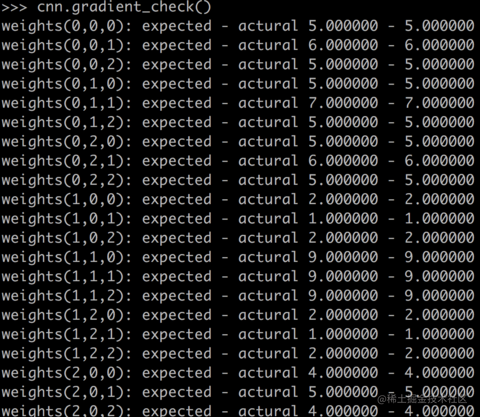### Max Pooling层的实现

max pooling层的实现相对简单，我们直接贴出全部代码如下：

class MaxPoolingLayer(object):
def __init__(self, input_width, input_height,
channel_number, filter_width,
filter_height, stride):
self.input_width = input_width
self.input_height = input_height
self.channel_number = channel_number
self.filter_width = filter_width
self.filter_height = filter_height
self.stride = stride
self.output_width = (input_width -
filter_width) / self.stride + 1
self.output_height = (input_height -
filter_height) / self.stride + 1
self.output_array = np.zeros((self.channel_number,
self.output_height, self.output_width))

def forward(self, input_array):
for d in range(self.channel_number):
for i in range(self.output_height):
for j in range(self.output_width):
self.output_array[d,i,j] = (
get_patch(input_array[d], i, j,
self.filter_width,
self.filter_height,
self.stride).max())

def backward(self, input_array, sensitivity_array):
self.delta_array = np.zeros(input_array.shape)
for d in range(self.channel_number):
for i in range(self.output_height):
for j in range(self.output_width):
patch_array = get_patch(
input_array[d], i, j,
self.filter_width,
self.filter_height,
self.stride)
k, l = get_max_index(patch_array)
self.delta_array[d,
i * self.stride + k,
j * self.stride + l] = \
sensitivity_array[d,i,j]复制代码

## 卷积神经网络的应用

### MNIST手写数字识别

LeNet-5是实现手写数字识别的卷积神经网络，在MNIST测试集上，它取得了0.8%的错误率。LeNet-5的结构如下：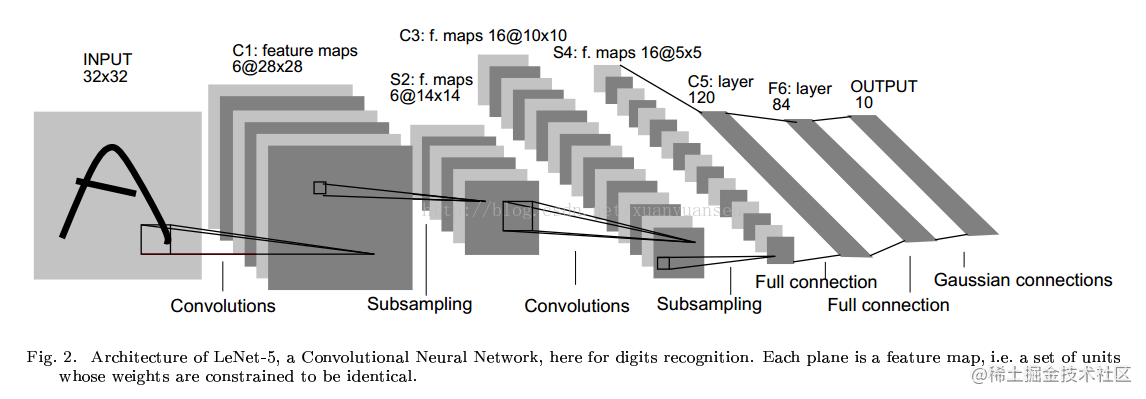## 小节## 参考资料

1. CS231n Convolutional Neural Networks for Visual Recognition
2. ReLu (Rectified Linear Units) 激活函数
3. Jake Bouvrie, Notes on Convolutional Neural Networks, 2006
4. Ian Goodfellow, Yoshua Bengio, Aaron Courville, Deep Learning, MIT Press, 2016